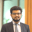Trusted answers to developer questions
Trusted Answers to Developer Questions

Related Tags

precision
average precision
object detection

# What is the mean average precision in object detection?Rana Abdul Muneem

Grokking Modern System Design Interview for Engineers & Managers

Ace your System Design Interview and take your career to the next level. Learn to handle the design of applications like Netflix, Quora, Facebook, Uber, and many more in a 45-min interview. Learn the RESHADED framework for architecting web-scale applications by determining requirements, constraints, and assumptions before diving into a step-by-step design process.### What is object detection?

Object detection is a method of recognizing and locating instances of an object from an image or video. Mean average precision (mAP) is a metric used to measure the accuracy of object detection models.

It is a value between $0–1$, with higher scores representing a more accurate model.

The following formula describes it:

In the formula above, $N$ is the total number of classes, and $AP_i$ is the average precision of class $i$. In simple terms, mAP is the average of average precisions across all classes.

To understand the calculation of mAP for object detection, we must first explore intersection over union, precision-recall curve, and average precision.

### Intersection over union (IOU)

Intersection over union (IOU) is a metric used to measure the accuracy of a bounding boxAn imaginary rectangle that contains an object. predicted by an object detector. The actual bounding box is the desired box that we set ourselves. The following image gives examples of actual and predicted bounding boxes.

Bounding boxes for an object detection model

IOU is the extent to which the predicted bounding box overlaps with the actual bounding box. It is a value between $0–1$.

An IOU is calculated by:

A visual representation of the IOU formula
1 of 2

IOU scores are converted into positive or negative predictions using a threshold. A typical threshold is $0.5$, meaning that samples with an IOU $\geq 0.5$ are labeled as positive matches while samples with IOU $< 0.5$ are labeled as negative matches.

The following table highlights how samples are categorized.

Evaluating Metrics
 True positve (TP) False positive (FP) False negative (FN) The predicted class is correct, and IOU is greater than or equal to the threshold. The IOU score is less than the threshold (less than 0.5) or multiple bounding boxes have been predicted. The IOU score was greater than or equal to 0.5 but the prediction was made for the wrong class.

The training data is assumed to have some objects present in each image. As a result, true negatives (TN) are not considered.

### Precision-recall curve

A precision-recall curve is a graph that shows the tradeoff between precision and recall.

A precision-recall curve can be plotted by following these steps:

1. Passing dataset of images to the object detection model.
2. Sorting results based on the received confidence scoresA value between 0–1 indicating the models certainty in its prediction..
3. Determining if the prediction was TP, FP, or FN.
4. Calculating ranked precisionPrecision for top k sorted results and ranked recallRecall for top k sorted results.

To understand the calculations in each step, let's consider an object detection model that can classify dogs. Let's suppose that the dataset contains only four images of dogs.

For each image, the model returns a bounding box, a predicted class, and a confidence scoreThe model's certainty in predicting the class for the predicted class. In the case of multiple predictions, we arbitrarily choose the prediction with the highest confidence. The bounding box and predicted class have been omitted for clarity.

The confidence score is returned for each image
1 of 7

The resultant values generate the following curve:

The precision-recall curve generated for our object detection model

### Average precision

Average precision is the area under the generated precision-recall curve. However, it is common practice to first smooth out the zig-zag pattern.

Using interpolated precisions (IP)The maximum precision for each unique recall. can smooth the curve. The calculation of IPs is as follows:

Identifying unique recall values
1 of 2

This results in the following graph:

Using IPs to smooth the precision-recall curve

There are many different variations for calculating AP from the precision-recall curve. The Pascal VOC challenge uses an 11-point interpolation.

1. The recalls are divided into 11 points (0 to 1.0 with a step size of 0.1).
2. We note the precision values at these 11 recall points.
3. AP is the average of these noted precision values.

In the equation above, $r$ takes on the 11 values, and $IP(r)$ is the interpolated precision at $r$ as read from the graph.

For the ongoing example, AP is calculated below:

### Mean average precision

The calculation for AP is repeated for each class of the object detector. The mAP for the model is the average of calculated APs.

Calculation of mAP across three classes

RELATED TAGS

precision
average precision
object detection

CONTRIBUTORRana Abdul Muneem